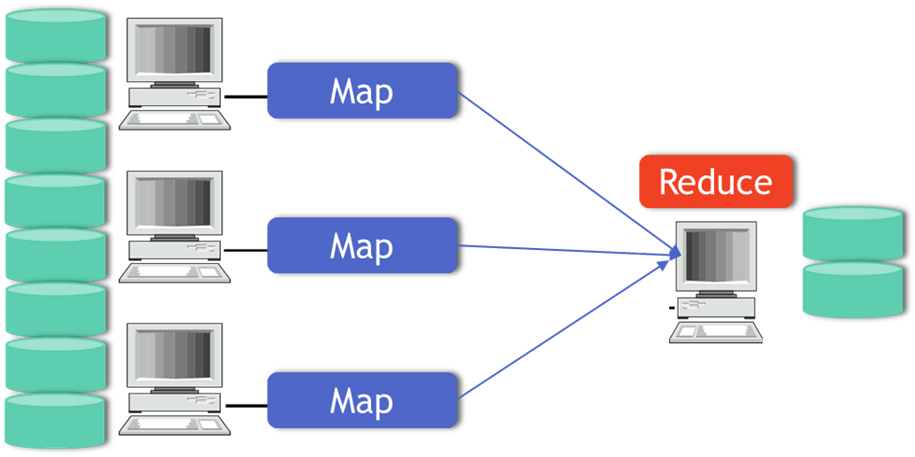• No products in the cart.

# 301.1.5-MapReduce and Network Programming

• Dividing The Overall Problem into Smaller Pieces
• Let us suppose we just want to simply find the number of lines or let’s say the data is from facebook, in a particular day how many likes are generated in facebook, that is what we want.
• Thus we have the huge data set of all the ‘likes’, which will be in TB’s or PB’s, we divide the data into smaller pieces, let’s say each row in that data set represents one ‘like’ or each row represents one activity i.e., if we want to count the number of activity we can divide the whole data into smaller pieces, put them on all these lower end computers, now overall problem is, we want to count the number of activities.
• We can divide this overall object also into smaller pieces. So we first count the activities in computer number one, whatever is the data on split one or data chunk 1, we can calculate the number of rows or we can calculate the number of activities, we can individually calculate the number of activities on all of these systems and we can do that parallely also and then once all these systems have locally calculated the number of rows or the number of activities, we can simply add them up later to get the final answer.
• Now, we divide data and store them locally and then on each of the data, we run the task and this is called map.
• Thus, on local systems at the map level or at very low level, we are calculating something, that is called map.
• Now, once we get output of the map then finally collate the results from local machines.
• Let us take a simple three Node Cluster, 3 nodes – three computers, so we do a whole dataset into computer 1 , 2 and 3.
• Then we take data chunk 1, 2 and 3 and the assign then to the 3 computers to work in parallel to calculate the number of row, andthis is called map.
• Once we have the result of all these maps, then we can calculate REDUCE that is nothing but the sum of all these rows.
• Thus, this is nothing but the distributed computing model.
• We can process bigdata in a parallel programming/distributed computing model.
• This is also known as MapReduce programming.### Map Reduce and Network Programming

#### MapReduce: Programming Model

• MapReduce:
• Map(the local/low level computation)
• We go to each and every data set and data chunk wherever it is and calculate something. The output of the map is the input of the reduce.
• Reduce(the collation of map results)
• Reduce will take this output, calculate something over it and then finally give you the result.
• Thus we will be processing the data using special map() and reduce() functions.

Map(): – The map() function is called on every item in the input and emits a series of intermediate key/value pairs(Local calculation). – All values associated with a given key are grouped together.

Reduce(): – The reduce() function is called on every unique key, and its value list, and emits a value that is added to the output(final organization).

• Since, we can’t handle the huge data set or big data using normal computers or conventional tools, we are making use of distributed computing.
• You distribute the data first and then you split the overall problem in such a way that you can code it in a MapReduce format, which is called the distributed computing.

22nd March 2018

### 0 responses on "301.1.5-MapReduce and Network Programming"

Statinfer Software Solutions LLP

Software Technology Parks of India,
NH16, Krishna Nagar, Benz Circle,